The electromagnetic wave travelling in space is the combination of both electric and magnetic fields. If we have a harmonic time varying source, it produces harmonic space varying waves. The E field producing H field shifted by 90 o and this H field in turn produces E field shifted by 90 o. Therefore the electric and magnetic fields both are perpendicular to each other and this E and H fields shifts continuously, which results the EM wave. If electric field travels in x-direction and magnetic field travels in y-direction, then the resulting EM wave travels in z-direction. The ratio of electric field strength to magnetic field strength is defined as characteristic impedance of the medium.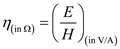For any medium the characteristic impedance is expressed in E and H field constants as follows:For a free space EM wave propagation,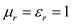. Hence,Substitute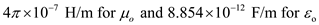.Therefore, characteristic impedance of free space is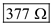.
The difference between power and power density: In lumped electric circuits power can be expressed as shown below.Here,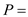power in watts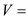voltage in volts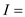current in amps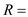resistance in ohms If you know any two parameters of V, I, and R then you can determine power of across an element in the circuit. The power density is nothing but power carried in the direction of propagation with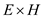indicating power per unit area. It is also called as instantaneous pointing vector. Here, the area is expressed in square meters and the power is expressed in watts. Therefore power density is expressed in watt/meter square. The power density of an electromagnetic wave is expressed as,Here,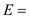electric field strength in V/m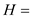magnetic field strength in H/m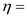impedance of the medium in ohms Since the power is transmitted in space and the isotropic radiator radiates equally in all directions, the power density in watts per square meters, would be the ratio of total power and the surface area of the sphere. Hence, The power density of a radiating element can be expressed as,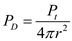……. (1) Here,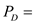power density in watt per square meter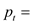total power in watts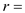distance from the antenna in meters. From equation (1), it is clear that the power density is inversely proportional to square of distance from the antenna. Hence power density decreases by increasing square of distance from the radiating element. Thus, the power density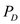decreases by the square of distance from the source.